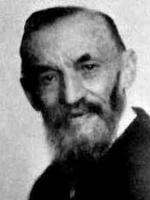# 引言

$1+1=2$ 这个等式是无人不知，无人不晓，基本上只要有 $3-4$ 岁的学习水平就应该充分了解这个等式，但是我们却从来没有想过它的来源是什么。

# 明确定理• $0$ 是自然数；
• 每一个确定的 $a$（$a\in \mathbb{N}$），都有确定的后继数 $a’,a’’$ 等也是自然数（数 $a$ 的后继数 $a’$ 等于它的后一个整数，也就是 $a’=a+1$）；

• $0$ 不是任何自然数的后继数；

• 不同的自然数有不同的后继数，如果自然数 $b,c$ 的后继数都是自然数 $a$，那么 $b=c$；

• 设 $S⊆\mathbb{N}$，且满足 $2$ 个条件：

• $0 \in S$，
• 如果 $n \in S$，那么 $n’ \in S$，

则 $S$ 包含全体自然数的集合，即 $S=\mathbb{N}$。

# 一些自然数运算的性质与定义

## 加法的定义

• $∀m\in \mathbb{N},0+m=m$；
• $∀m,n \in \mathbb{n},n’+m=(n+m)’$。